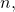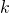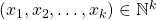# Number of tuples with a given least common multiple

K. Siddharth Choudary and A. Satyanarayana Reddy
Notes on Number Theory and Discrete Mathematics
Print ISSN 1310–5132, Online ISSN 2367–8275
Volume 26, 2020, Number 2, Pages 53—60
DOI: 10.7546/nntdm.2020.26.2.53-60

## Details

### Authors and affiliations

K. Siddharth ChoudaryDepartment of Mathematics, Shiv Nadar University
India-201314

A. Satyanarayana ReddyDepartment of Mathematics, Shiv Nadar University
India-201314

### Abstract

In this paper, for a given natural numberwe count the number of-tupleswith certain conditions such that lcmIn the process, we derived different arithmetic functions.

### Keywords

• Arithmetic function
• Multiplicative function
• Least common multiple
• Stirling numbers of the second kind

• 11A41
• 11A51
• 11A25

### References

1. Apostol, T. M. (1976). Introduction to Analytic Number Theory, Springer-Verlag.
2. Bagdasar, O. (2014). On some functions involving the lcm and gcd of integer tuples, Scientific Publications of the State University of Novi Pazer, Ser. A: Appl. Math. Inform. and Mech., 6 (2), 91–100.
3. Burton, D. M. (2010). Elementary Number Theory, McGraw-Hill Higher Education, 7th edition.
4. Djamel, B. A., & Özer, Ö. (2019). On a sequence formed by iterating a divisor operator, Czechoslovak Mathematical Journal, 69 (4), 1177–1196.
5. Erdős, P. (1964). On the multiplicative representation of integers, Israel J. Math., 2, 251–261.
6. Hardy, G. H., & Wright, E. M. (1979). An Introduction to the Theory of Numbers,
5th edition, Oxford University Press, Oxford.
7. Jones, G. A., & Jones, J. M. (1998). Elementary Number Theory, Springer Undergraduate Mathematics Series.
8. Nathanson, M. B. (1987). Multiplicative representations of integers, Israel J. Math., 57, 129–136.

## Cite this paper

Siddharth Choudary, K., & Satyanarayana Reddy, A. (2020). Number of tuples with a given least common multiple. Notes on Number Theory and Discrete Mathematics, 26 (2), 53-60, doi: 10.7546/nntdm.2020.26.2.53-60.